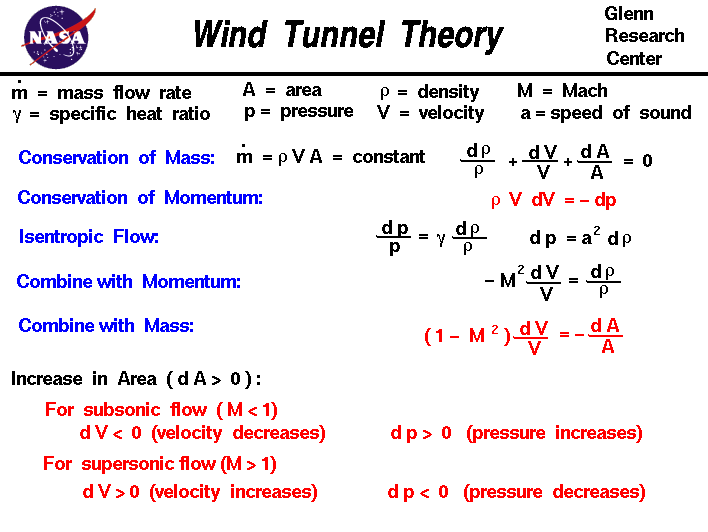+ Text Only Site
+ Non-Flash Version
+ Contact GlennAerodynamicists use wind tunnels to test models of proposed aircraft. In the tunnel, the engineer can carefully control the flow conditions which affect forces on the aircraft. By making careful measurements of the forces on the model, the engineer can predict the forces on the full scale aircraft. And by using special diagnostic techniques, the engineer can better understand and improve the performance of the aircraft. Wind tunnels are designed for a specific purpose and speed range and there is a wide variety of wind tunnel types and model instrumentation. The model to be tested in the wind tunnel is placed in the test section of the tunnel. The speed in the test section is determined by the design of the tunnel. The choice of speed range affects the design of the wind tunnel due to compressibility effects. On this slide we derive the equations which explain and describe why a supersonic flow accelerates in the diffuser section of the tunnnel while a subsonic flow decelerates in a divergent duct. We begin with the conservation of mass equation: mdot = rho * V * A = constant where mdot is the mass flow rate, rho is the gas density, V is the gas velocity, and A is the cross-sectional flow area. If we differentiate this equation, we obtain: V * A * drho + rho * A * dV + rho * V * dA = 0 divide by (rho * V * A) to get: drho / rho + dV / V + dA / A = 0 Now we use the conservation of momentum equation: rho * V * dV = - dp and an isentropic flow relation: dp / p = gam * drho / rho where gam is the ratio of specific heats. This is Equation #10 on the page which contains the derivation of the isentropic flow relations We can use algebra on this equation to obtain: dp = gam * p / rho * drho and use the equation of state p / rho = R * T where R is the gas constant and T is temperature, to get: dp = gam * R * T * drho gam * R * T is the square of the speed of sound a: dp = (a^2) * drho Combining this equation for the change in pressure with the momentum equation we obtain: rho * V * dV = - (a^2) * drho V / (a^2) * dV = - drho / rho - (M^2) * dV / V = drho / rho using the definition of the Mach number M = V / a. Now we substitute this value of (drho /rho) into the mass flow equation to get: - (M^2) * dV / V + dV / V + dA / A = 0 (1 - M^2) * dV / V = - dA / A This equation tells us how the velocity V changes when the area A changes, and the results depend on the Mach number M of the flow. If the flow is subsonic then (M < 1) and the term multiplying the velocity change is positive ([1 - M^2] > 0). Then an increase in the area (dA > 0 ) produces a negative increase (decrease) in the velocity (dV < 0). For a subsonic wind tunnel, the flow downstream of the test section will decelerate and stay subsonic. For a supersonic flow (M > 1) the term multiplying velocity change is negative ([1 - M^2] < 0). Then an increase in the area (dA > 0) produces an increase in the velocity (dV > 0). This is exactly the opposite of what happens subsonically. Why the big difference? Because in supersonic (compressible) flows, both the density and the velocity are changing as we change the area in order to conserve mass. For subsonic (incompressible) flows, the density remains fairly constant, so the increase in area produces a decrease in velocity to conserve mass. But in supersonic flows, there are two changes; the velocity and the density. The equation: - (M^2) * dV / V = drho / rho tells us that for M > 1, the change in density is much greater than the change in velocity. To conserve both mass and momentum in a supersonic flow, the velocity increases and the density decreases as the area is increased. Navigation ..Beginner's Guide Home Page+ Inspector General Hotline + Equal Employment Opportunity Data Posted Pursuant to the No Fear Act + Budgets, Strategic Plans and Accountability Reports + Freedom of Information Act + The President's Management Agenda + NASA Privacy Statement, Disclaimer, and Accessibility CertificationEditor: Nancy Hall NASA Official: Nancy Hall Last Updated: May 13 2021 + Contact Glenn